Back to AIHPA
Australian High Power RifleTargets

Some of the most common targets used are outlined below.  This is not a complete list, but are the typical, and most commonly, used targets for AIHPA meets.

Scaled/reduced size targets allow for shooting at distances closer than specified for the course of fire.  Further information on these targets may be found in the NRA HPR rulebook.

 X-Ring Decimal Targets 200 Target SR (Short Range - Full Size)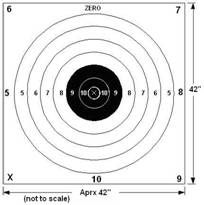X =       3”    10 =      7”    9 =       13” (Aiming mark)    8 =       19”    7 =       25”    6 =       31”    5 =       37” Remainder target value is zero. O/All approx 40" x 42" 300 Target SR-3 (Short Range - Full Size) & 400 for reduced from 600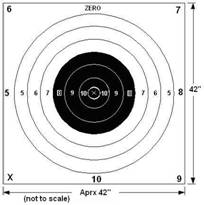X =       3”    10 =      7”    9 =       13”    8 =       19” (Aiming mark)    7 =       25”    6 =       31”    5 =       37” Remainder target value is zero. O/All approx 40" x 42" 600 Target MR-1 (Mid Range - Full Size)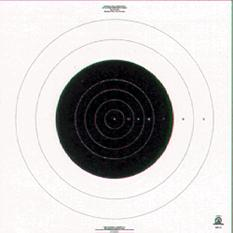X =       6”    10 =      12”    9 =       18”    8 =       24”    7 =       36” (Aiming mark)    6 =       48”    5 =       60”    Remainder target value is zero.    O/All approx 6'x6' 200 Target MR-52 (Short Range) 600 reduced to 200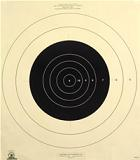X =     1.79”    10 =    3.79”    9 =     5.79”    8 =     7.79”    7 =     11.79” (Aiming mark)    6 =     15.79”    5 =     19.79” Remainder target value is zero. O/All approx 21" x 24" 200 Target SR-42 (Short Range) 300 reduced to 200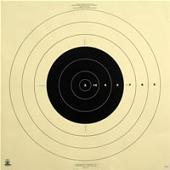X =     1.9”    10 =   4.56”    9 =     8.56”    8 =     12.56” (Aiming mark)    7 =     16.56”    6 =     20.59”    5 =     24.56” Remainder target value is zero. O/All approx 28" x 28" 300 Target MR-63 (Mid Range) 600 reduced to 300X =       2.85”    10 =      5.85”    9 =       8.85”    8 =       11.85”    7 =       17.85” (Aiming mark)    6 =       23.85”    5 =       29.85” Remainder target value is zero. O/All approx 35" x 35" Note:  For Australian Clubs, these X-ring targets are available from AIHPA for a nominal fee.  Contact AIHPA for quantities, costs and shipping arrangements.  See Merchandise for pricing.. Figure Targets - NRAA & SSAAType B 4x4 with Figure 12. Type B represents 8" (200mm) scoring ring convention.    V =     6”    5 =     8”    4 =     16”    3 =     32”    2 =     48” Remainder target value is zero. O/All approx 4' x 4'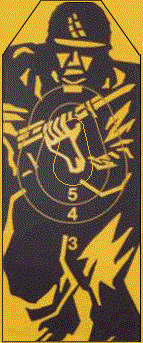Figure 11 (typical rapid target)              W         H    V =     6”        9.5"    5 =     9.5”     13"    4 =     14”      17.5"    3 =     Remainder of target,     O/All approx 18.5" x 45.5"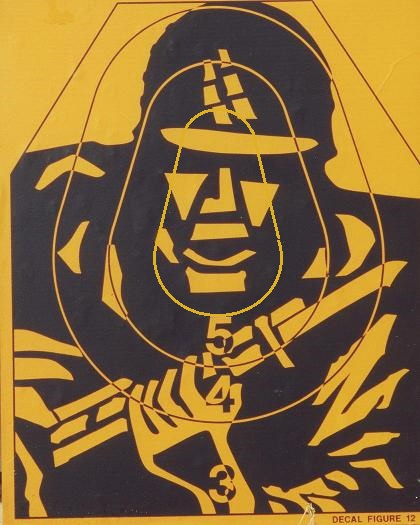Figure 12 (typical snap target)              W         H    V =     6”        9.5"    5 =     9.5”     13"    4 =     14”      17.5"    3 =     Remainder of target,     O/All approx 18.5" x 23"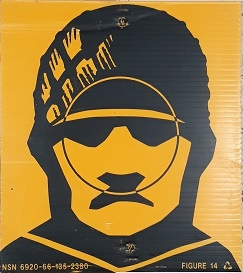Figure 14 (typical sniper target) Target size: 255 Wide 285mm High Scoring: - Circle 125mm = 5 points. - Remainder of image = 3. - On target miss = 0.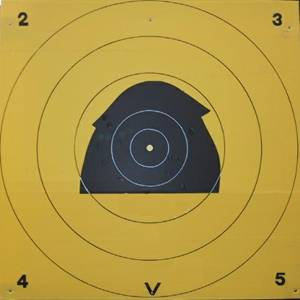Type C 4x4 with SSAA aiming mark.  Type C represents 6" (150mm) scoring rings.    V =     6”    5 =     12”    4 =     24”    3 =     36”    2 =     48” Remainder target value is zero. O/All approx 4' x 4' Type C SSAA target available from SSAA Queensland. Type B, Fig 11 and 12 targets, some available from us. email us if any interest.